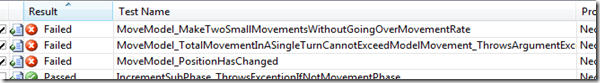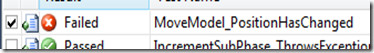# Learning Unit Testing V – Refactoring Mistakes

I implied in the last post that I had made a mistake when I moved the MoveModel code into the Model class from the MovementManager class. Now, tests on the MovementManager class are actually testing functionality in the Model class. I also discovered that I am not properly testing the ‘GetDistanceFrom’ method with known data at all. This will have to be corrected.

Since I already have Model implementing an IModel interface, it’ll be easy enough to mock the Model part in the MovementManager. To see what tests are reaching into Model.MoveModel, I can just alter that method to throw an exception and see which tests fail.Since this functionality has been moved to the Model class, these tests should actually be made against the Model class itself. Simple? Yes, but incorrect, as I found out next – it still fails on one condition and checking further, I don’t believe it’s checking the other one correctly. The validation of whether or not a model may make a move (ie, the distance is still under it’s Movement rate) is done in the MovementManager. My two options at this point are: move the validation back to the MovementManager and do a lot of mocking around the Model, or to move that validation into the Model as a ValidateMove method. Then the movement manager really becomes a mere progress tracker for the Movement phase… but I think that’s probably the best idea in this situation. Just one more test to move over (MoveModel_CannotMoveFurtherThanModelsMovement), then the GetDistanceFrom method needs to be tested. I think the following cases will need to be covered:

• Full three-dimensional movement (all values change)
• Two-dimensional movement (one value doesn’t change)
• One-dimensional movement (only one value does change)
• Cover reverse cases of each of the above (so the direction is backwards)

This test (worked on with a calculator and doodles to prove my working), however:

```[TestMethod]
{
Model testModel = new Model();
testModel.PositionX = 1;
testModel.PositionY = 3;
testModel.PositionZ = 4;
double result = testModel.GetDistanceFrom(2, 5, 7);
Assert.AreEqual(3.74, Math.Round(result, 2));
}```

Shows me that either my test calculation is wrong or my original working is wrong. In the GetDistanceFrom method, this line

`distance = Math.Sqrt(Math.Sqrt((differenceX * differenceX) + (differenceY * differenceY)) + (differenceZ * differenceZ));`

Should actually be

`distance = Math.Sqrt((differenceX * differenceX) + (differenceY * differenceY) + (differenceZ * differenceZ));`

So I got the calculation wrong. Now the test passes, but another one breaks.This is because that movement calculation now requires the model to move further than it’s allowed distance. Fixing that test’s values (from 3, 4, 5 to 2, 2, 2) and adding in the following tests:

```[TestMethod]
{
Model testModel = new Model();
testModel.PositionX = 1;
testModel.PositionY = 3;
testModel.PositionZ = 4;
double result = testModel.GetDistanceFrom(1, 5, 7);
Assert.AreEqual(3.61, Math.Round(result, 2));
}
[TestMethod]
{
Model testModel = new Model();
testModel.PositionX = 1;
testModel.PositionY = 3;
testModel.PositionZ = 4;
double result = testModel.GetDistanceFrom(1, 3, 7);
Assert.AreEqual(3, Math.Round(result, 2));
}
[TestMethod]
{
Model testModel = new Model();
testModel.PositionX = 2;
testModel.PositionY = 5;
testModel.PositionZ = 7;
double result = testModel.GetDistanceFrom(1, 3, 4);
Assert.AreEqual(3.74, Math.Round(result, 2));
}
[TestMethod]
{
Model testModel = new Model();
testModel.PositionX = 1;
testModel.PositionY = 5;
testModel.PositionZ = 7;
double result = testModel.GetDistanceFrom(1, 3, 4);
Assert.AreEqual(3.61, Math.Round(result, 2));
}
[TestMethod]
{
Model testModel = new Model();
testModel.PositionX = 1;
testModel.PositionY = 3;
testModel.PositionZ = 7;
double result = testModel.GetDistanceFrom(1, 3, 4);
Assert.AreEqual(3, Math.Round(result, 2));
}```

Gives me 19 passes out of 19 tests. Excellent – I was expecting some of the 0-distance values being squared and rooted to throw some sort of calculation exception in .Net, but I can get along with this result.

This leaves me with my refactoring mess cleaned up, a calculation error solved, a method I somehow missed tests for covered properly, and all ready to tackle the last part of page 10 – a much longer page than I had initially expected. “Ooh look”, said the me at the beginning of the week, “half the page is picture and prose, and the other half is mostly descriptive. One or two posts to talk about design ideas and I’ll be on page 20 in a fortnight!” Isn’t hindsight awesome.

This is a learning project I am documenting in order to teach myself TDD and unit testing – as I publish this, I have already written many parts in advance but I want to know what I’m doing wrong! If you see improvements or corrections, either to the code or the process, please leave a comment and let me know! Introduction to this project can be found here.